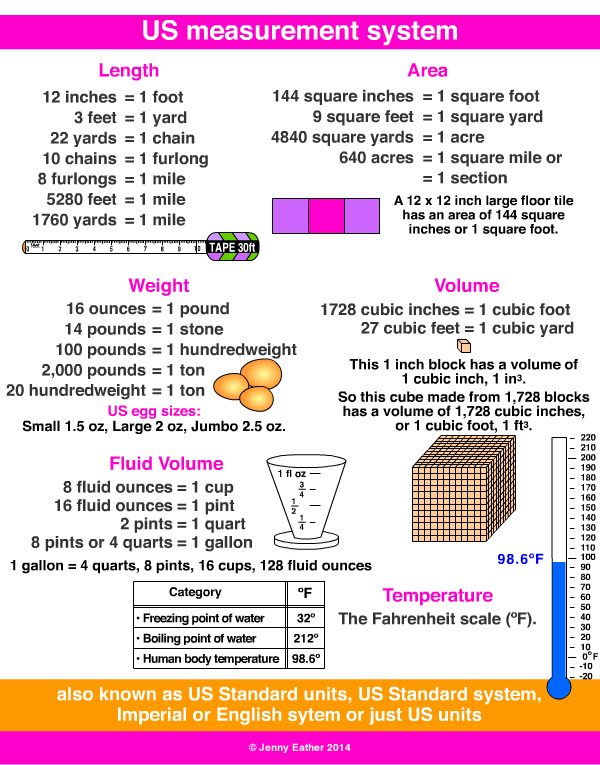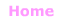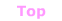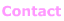Aa Bb Cc Dd Ee Ff Gg Hh Ii Jj Kk Ll Mm Nn Oo Pp Qq Rr Ss Tt Uu Vv Ww Xx Yy Zz

Uu

US measurement system

• may also be called U.S. Customary Measures, US Standard units,
US Standard System, Imperial or English system or just US units.

EXAMPLES:US Standard Units ~ Length

12 inches = 1 foot
3 feet = 1 yard
22 yards = 1 chain
10 chains = 1 furlong
8 furlongs = 1 mile
5280 feet = 1 mile
1760 yards = 1 mile

US Standard Units ~ Area

144 square inches = 1 square foot
9 square feet = 1 square yard
4,840 square yards = 1 acre
640 acres = 1 square mile

US Standard Units ~ Volume

1728 cubic inches = 1 cubic foot
27 cubic feet = 1 cubic yard

US Standard Units ~ Fluid Volume

8 fluid ounces = 1 cup
16 fluid ounces = 1 pint
2 pints = 1 quart
4 quarts = 1 gallon

1 gallon = 4 quarts, 8 pints, 16 cups, 128 fluid ounces

US Standard Units ~ Weight

437.5 grains = 1 ounce
16 ounces = 1 pound
14 pounds = 1 stone
100 pounds = 1 hundredweight
20 hundredweight = 1 ton
1 ton = 2000 pounds

Overseen by NIST the US National Institute of Standards and Technology© Jenny Eather 2014. All rights reserved.